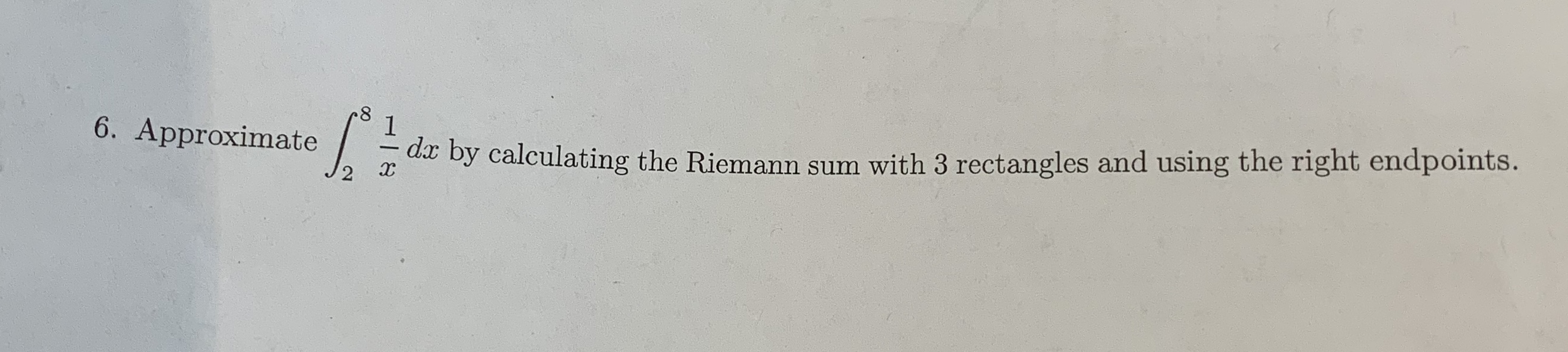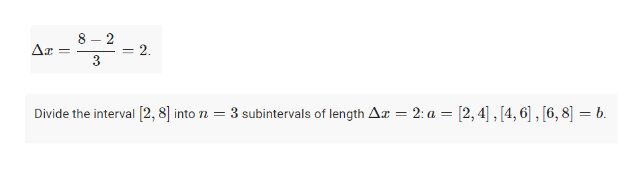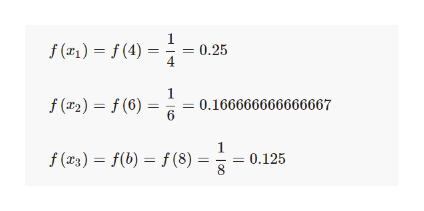# 816. Approximateax by calculating the Riemann sum with 3 rectangles and using the right endpoints.

Question
2 viewshelp_outlineImage Transcriptionclose8 1 6. Approximate ax by calculating the Riemann sum with 3 rectangles and using the right endpoints. fullscreen
check_circle

Step 1

We have that a=2, b=8, n=3. Therefore, del x= 2

Then find the three intervals.help_outlineImage Transcriptionclose8-2 2 3 subintervals of length Ar = 2: a = [2,4],[4,6], [6,8] = b Divide the interval 2, 8into n = fullscreen
Step 2

Now, we evaluate the function at ...help_outlineImage Transcriptionclosef () =(4) 0.25 f (2)= (6) 0.166666666666667 6 f () f(b) f(8) 0.125 fullscreen

### Want to see the full answer?

See Solution

#### Want to see this answer and more?

Solutions are written by subject experts who are available 24/7. Questions are typically answered within 1 hour.*

See Solution
*Response times may vary by subject and question.
Tagged in

### Calculus